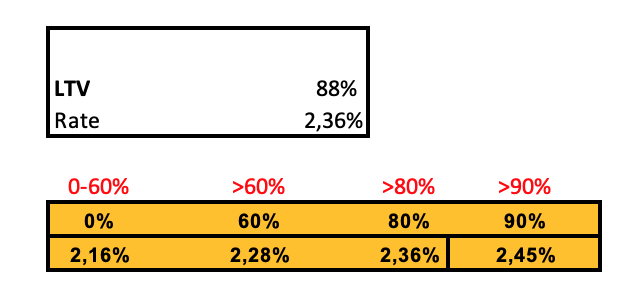# Converting an index match setup to Tadabase

Hi, I am new here. Is it possible to setup a database table in such a way to get the folllowing result: input LTV → reflect interest %? The screenshot is from Google Sheets.There is no native way of doing this, but I’m sure we can find a way.

Here’s a few ideas:

A) If you’re working with just a few basic values, you can use an equation field with IF statements.

B) If it’s somewhat more complex, you can use some JavaScript Pipe:

C) You can also the Rest API to lookup based on a single value, not exactly like Index and Match, you can see similar post here:

D) If you need a true Index and Match you can turn your a Google Spreadsheet into a standalone database and run a request against it in conjuction with a Pipe. This is complex.

I’ll make a video shortly to explain how to do this. It’s complex and will require advanced knowledge in Pipes and Google Sheets.

1 Like

@slimpens, just made you a video with the full walk through.

Video:

Here’s the doc I’m using:

Google App Script Code:

``````function doGet(e) {

var INCOME=e.parameter.INCOME
var RATE=e.parameter.RATE

var response=findRow(INCOME, RATE)
return ContentService.createTextOutput(JSON.stringify(response) ).setMimeType(ContentService.MimeType.JSON);
}
function findRow(INCOME, RATE){
const sheet=ss.getSheets()
const data=sheet.getDataRange().getValues()
var result=[]

data.forEach(function(row){
if(row==INCOME) return result=row
})

var val1;
for(var i=0; i<header.length; i++) {
Logger.log(header[i]+ ' ' + RATE)
if(header[i] == RATE) {
val1=result[i]
return;
}
}

if (val1 == undefined) {
for(var i=1; i<header.length-1; i++) {
if (header[i] < RATE && header[i+1] > RATE) {
if ( Math.abs(header[i]-RATE)  > Math.abs(header[i+1]-RATE) ) {
val1 = result[i+1];
} else {
val1 = result[i];
}
}
}
}
return (val1)
}